## Systems of Equations Solved Graphically

Graphs can be used to solve systems of equations. This method, however, usually allows only approximate solutions, whereas the algebraic method arrives at exact solutions.

##### Example 1

Solve the following system of equations graphically.

• (1)

x 2 + 2 y 2 = 10

• (2)

3 x 2y 2 = 9

Equation (1) is the equation of an ellipse. Convert the equation into standard form.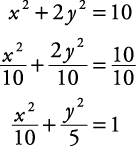The major intercepts are at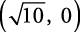and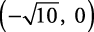, and the minor intercepts are atand.

Equation (2) is the equation of a hyperbola. Convert the equation into standard form.The transverse axis is horizontal, and the vertices are at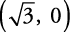and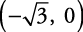, as shown in Figure 1.

The approximate answers areThe exact answers are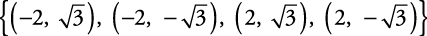Refer to Example for the algebraic approach to this problem; it gives the exact answers.